## 集合

### 集合的关系与运算

#### 集合间的关系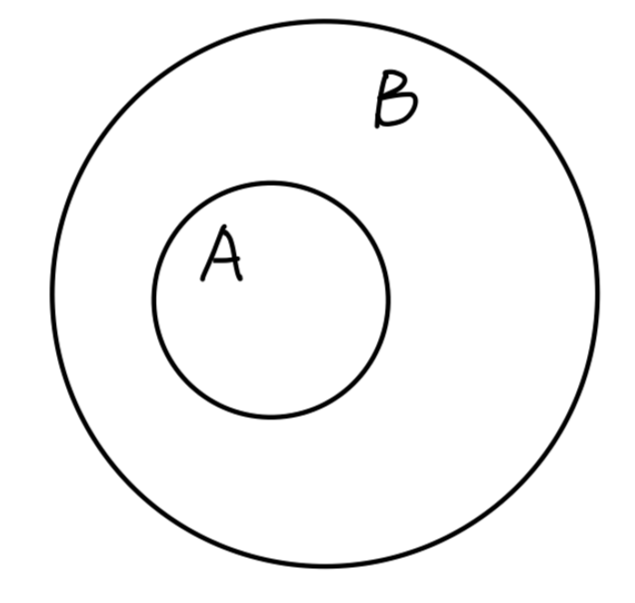Fig: $A$$B$ 的子集

$\forall\ a\in A,\ s.t.\ a\in B$

$A\subset B$

$A\subsetneq B$

#### 集合的运算

• 并集 (union)

$A\cup B\equiv \{x|x\in A\ or\ x\in B\}$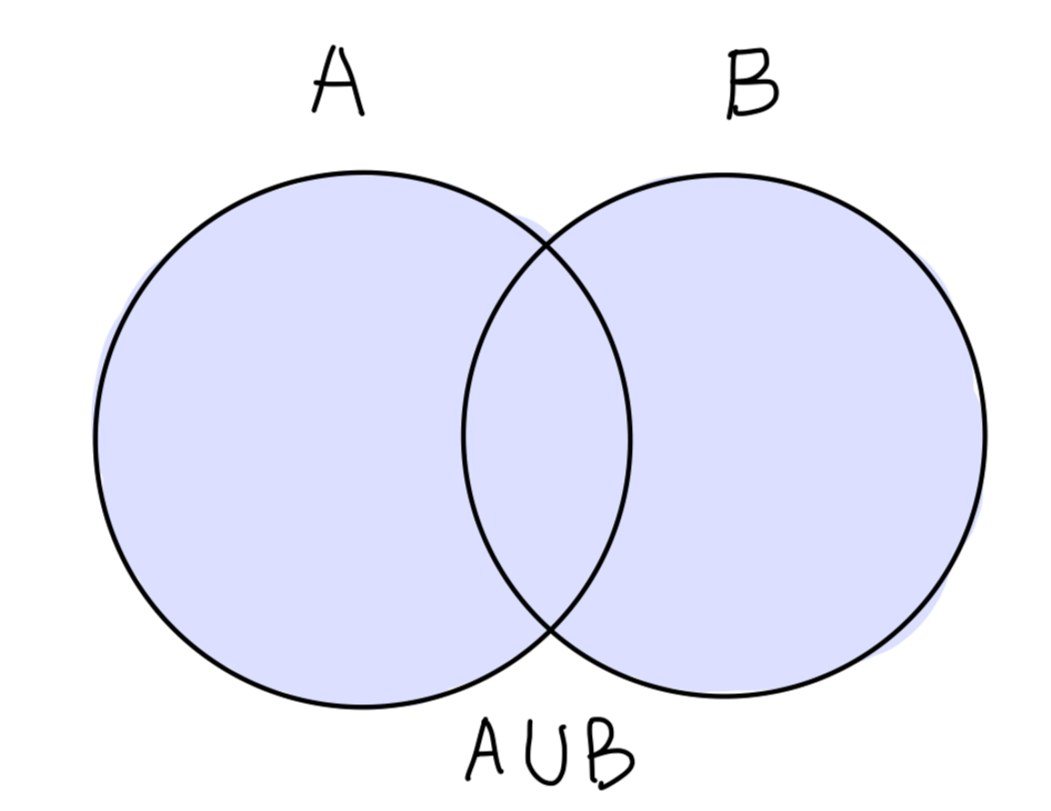Fig：$A$$B$ 的并集

• 交集 (intersection)

$A\cap B\equiv \{x|x\in A\ and\ x\in B\}$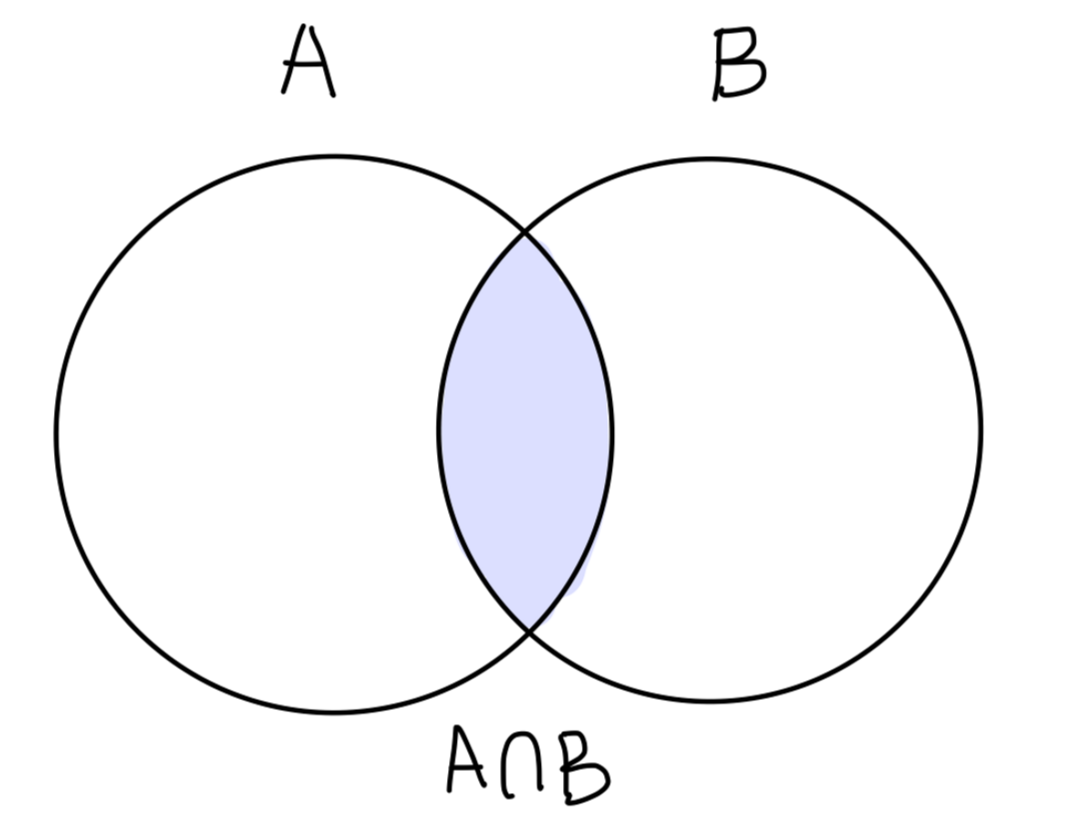Fig：$A$$B$ 的交集

$A \cap B = \empty$，称 $A,B$ 无交集 (disjoint)。

• 补集 (complement)
$A\subset U$$A$ 关于全集 $U$ 的补集为：
$\complement_{U}A\equiv\{x|x\notin A\ and\ x\in U\}$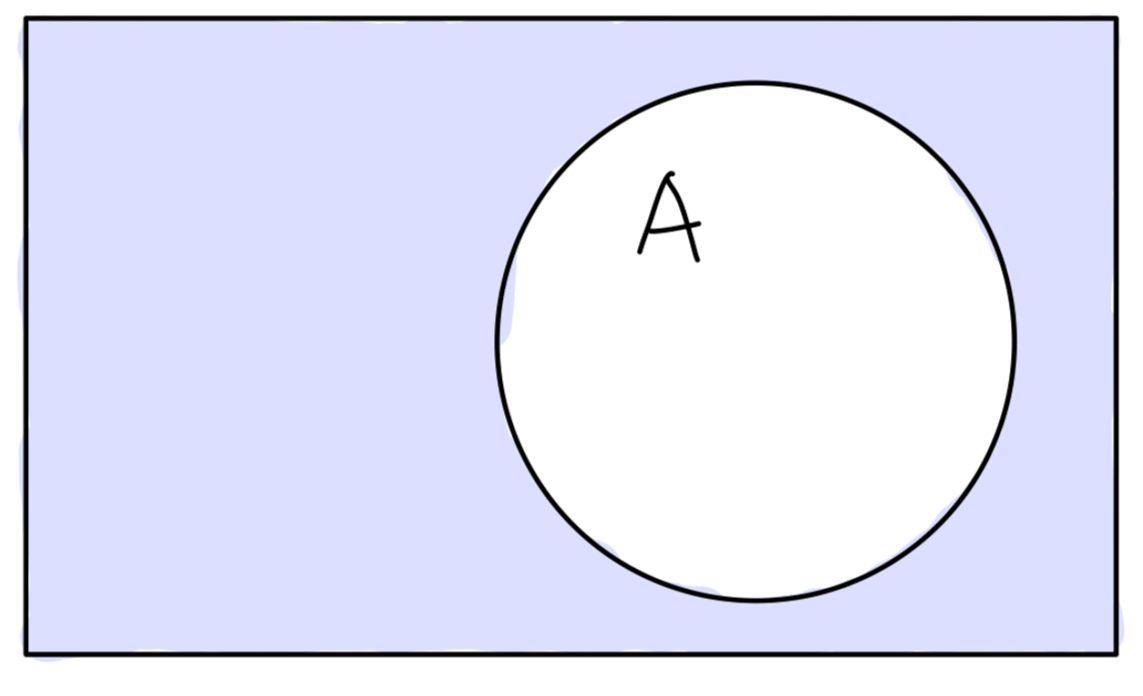Fig：$A$ 关于全集 $U$ 的补集

• 差集 (difference)
两个集合也可以相"减"。$A$$B$ 中的 相对补集 (差集) 定义为：

$A \setminus B \equiv \{x|x\in A \ and\ x\notin B \}$

$A\subset U$,$B\subset U$，则有：

$A \setminus B = A \cap \complement_{U} B$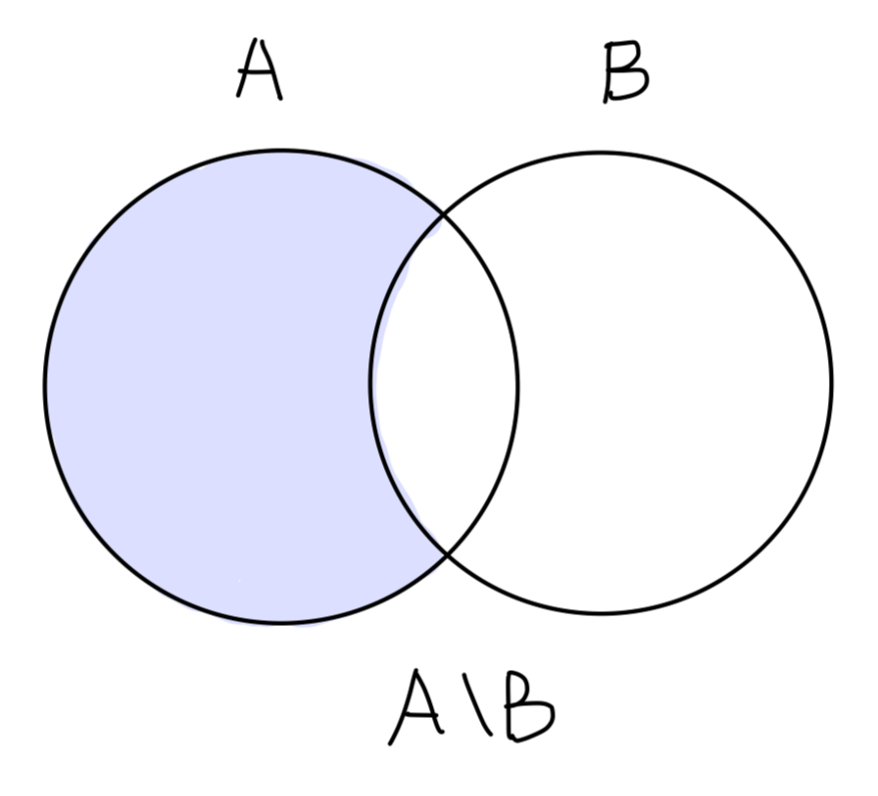Fig：相对补集 $A \setminus B$

$\complement_{U}A=U\setminus A$

• 对称差集 (symmetric difference)
$A \subset U, B \subset U$，则其对称差运算如下：

$A \vartriangle B \equiv (A − B) ∪ (B − A)$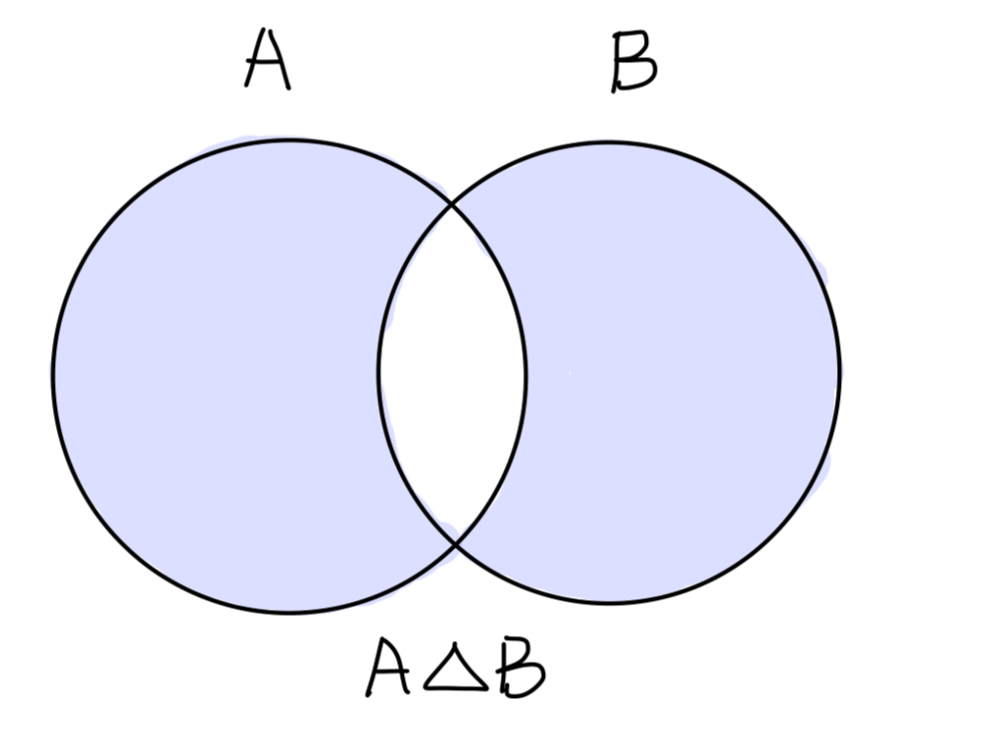Fig：对称差集 $A \vartriangle B$

• 集合的 卡氏积 (直积) (Cartisian product)

$X × Y \equiv \{(x,y)|x ∈ X,y ∈ Y \}$

• 幂集 (power set)
$X$ 的幂集为其所有子集的集合，记为 $2^{X}$

$2^X \equiv \{ A| A\subset X\}$

• 交换律

$X \cup Y = Y \cup X, X \cap Y = Y \cap X$

• 结合律

\begin{aligned} &(X \cup Y ) \cup Z = X \cup (Y \cup Z)\\ &(X \cap Y )\cap Z = X \cap (Y \cap Z)\\ \end{aligned}

• 分配律

\begin{aligned} (X\cap Y )\cup Z = (X\cup Z)\cap (Y \cup Z)\\ (X\cup Y )\cap Z = (X\cap Z)\cup (Y \cap Z)\\ \end{aligned}

• De Morgan 律

\begin{aligned} &X\setminus(Y \cup Z) = (X\setminus Y )\cap (X\setminus Z)\\ &X\setminus (Y \cap Z) = (X\setminus Y )\cup (X\setminus Z)\\ \end{aligned}

$Y,Z\subset X$

\begin{aligned} &\complement_{X} \setminus(Y \cup Z) = (\complement_{X}\setminus Y )\cap (\complement_{X}\setminus Z)\\ &\complement_{X}\setminus (Y \cap Z) = (\complement_{X}\setminus Y )\cup (\complement_{X}\setminus Z)\\ \end{aligned}

\begin{aligned} & \forall x(x\in X \setminus (Y\cup Z))\\ \Leftrightarrow & \forall x( (x\in X) \land (x\notin Y \cup Z))\\ \Leftrightarrow & \forall x( (x\in X) \land ((x\notin Y) \land (x\notin Z)))\\ \Leftrightarrow & \forall x( ((x\in X) \land (x\notin Y)) \land ((x\in X) \land (x\notin Z))))\\ \Leftrightarrow & \forall x( (x \in X \setminus Y) \land (x \in X \setminus Z))\\ \Leftrightarrow & \forall x( x \in (X \setminus Y) \cap (X \setminus Z))\\ \end{aligned}

$\forall x ((x\in X \setminus (Y\cup Z)) \Leftrightarrow ( x \in (X \setminus Y) \cap (X \setminus Z)))$

$X\setminus(Y \cup Z) = (X\setminus Y )\cap (X\setminus Z)$

## 映射

### 映射的基本概念

$A$$B$ 是两个非空集合，若对 $A$ 中的任一元素 $x$，依照某种规律 (或法则)$f$，恒有 $B$ 中的唯一确定的元素 $y$ 与之对应，则称对应规律 $f$ 为一个从 $A$$B$映射\$。记作：

$f:A\rightarrow B$

$f:x\mapsto y$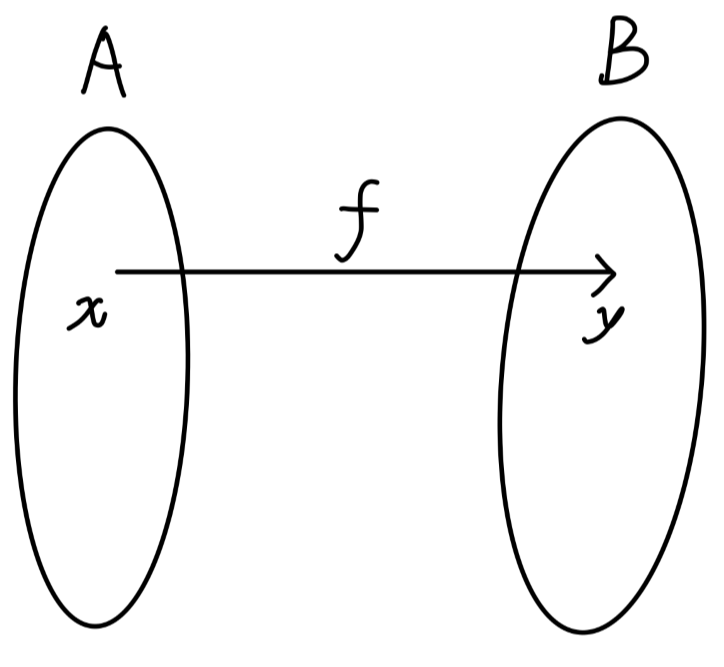### 单射、满射与双射

• 单射 (one-to-one mapping, injection)
对每一个 $y \in f(X)$，存在一个唯一的 $x \in X$ 使得 $f(x) = y$• 满射 (onto mapping, surgection)
对任意一个 $y \in Y$ 都有逆。• 双射 (bijection)
既是单射又是满射。### 映射的其他概念

• 复合映射 (composite mappings)
$f$$g$ 是两个映射：

$f : X \rightarrow Y ,\ g : Y \rightarrow Z$

• 映射的限制 (restriction)
映射 $f$ 在某个子集 $U$ 上的限制记为 $f|_ U$，对应法则不变而定义域发生了变化。即：

$f|_{U}: U \rightarrow Y,\ x \mapsto f(x)$

• 映射的扩张 (extension)
映射 $f$ 称为映射 $g$ 的扩张，若:
$D(f) \supset D(g)$，且对于任意 $x \in D(g)$，有 $f(x) = g(x)$
反过来有：

$g = f|_{U(g)}$

• 常映射 (constant mapping)
映射 $f$ 称为常映射，若:
$\forall x,x'\in D(f)$，有 $f(x) = f(x')$

• 恒等映射 (identity mapping)
映射 $f:X\rightarrow X,\ x \mapsto x$ 称为恒等映射. 通常记为 $\mathrm{Id}$。它显然是个双射。即：

$\mathrm{Id}_X: X\rightarrow X, x \mapsto x$

• 子集的 序列 (sequence)
集合 $X$ 的一些子集 $\{A_n \}$ 称为一个序列，若由它可构造一个映射

$\mathbb{N}\mapsto 2^X , n\mapsto A_n$

• 集合的 对等 (equipotent)（等势）
称集合 $X$$Y$ 对等，若存在一个从 $X$$Y$ 的双射。

• 可数集 (countable)
集合 $X$ 被称为可数集，若存在一个由 $X$$\mathbb{N}$ 的双射。（即 $X$$\mathbb{N}$ 对等）